## Monday, 13 October 2014

### Prime Venn diagrams

Last time I gave a rigorous proof that if a number, N, was composite then the N-set Venn diagram could not be rotationally symmetric. Further, as we have seen previously, the 2-, 3- and 5-set diagrams (reproduced below) do have rotational symmetric forms.
Surely, the evidence suggests that all Venn diagrams with prime numbers of sets have rotational symmetric forms, right?

Well, of course, "suggestion" isn't good enough for mathematicians. We demand logical proof more rigorous than any other science.

Surprisingly, this question has only been laid to rest relatively recently. In fact, up until 1992 some people thought that a rotational form of the 7-set diagram did not exist at all. The 7-set rotationally symmetric diagram was first created by Branko Grünbaum, whilst he was actually trying to disprove its existence!A 7-set rotational Venn diagram.
There the problem stayed until 1999, when Peter Hamburger introduced a new idea of how to generate such diagrams . Using his technique he pushed the bounds to 11 sets. Two examples of such diagrams can be found below.
Building on Hamburger's technique Jerrold Griggs, Carla Savage and Charles Killian demonstrated that it was possible to produce rotationally symmetric Venn diagrams whenever the number of sets is prime . Unfortunately, the proof of this claim is quite complex and far beyond my capabilities to convey in a simple and intuitive way. However, for those seeking more details, the reference can be found below.

So, the question was finally laid to rest. Or was it? As I originally stated when we first started discussing rotationally symmetric diagrams, mathematicians love to abstract any property they can. Hence, once a problem has been solved a mathematician will try to solve the problem once again under heavier constraints.

Thus, mathematicians are now looking for Venn diagrams that are "simple". A Venn diagram is simple if at all points there are at most two sets crossing each other. In the diagrams above the constructions are so complicated that at some points three or more sets cross each other at the same point.

The question of existence and how to create a simple rotationally symmetric Venn diagram is still open. However, in 2012 Khalegh Mamakani and Frank Ruskey produced the first example of a simple symmetric 11-Venn diagram . This has been reproduced below.
Zooming into the Venn diagram (below) we see the intricate details of all the curves passing through one another. Yet, by definition, we can be sure that there are only ever at most two sets crossing at each point.

As we have reached the edges of our current knowledge we come to the end of my posts regarding rotationally symmetric Venn diagrams. I hope you have enjoyed learning about these beautiful diagrams just as much as I have enjoyed creating them.
Next time, something completely different!
________________________________________________________________
________________________________________

 Doodles and Doilies, Non-Simple Symmetric Venn Diagrams. Peter Hamburger.
 Venn Diagrams and Symmetric Chain Decompositions in the Boolean Lattice. Jerrold Griggs, Charles E. Killian, Carla D. Savage.
 A New Rose: The First Simple Symmetric 11-Venn Diagram. Khalegh Mamakani and Frank Ruskey.

## Monday, 22 September 2014

### Primes, composites and rotationally symmetric Venn diagrams

Last time I boldly stated that if a Venn diagram is made up of $N$ sets, where $N$ is a composite number, i.e. not prime , then the Venn diagram can never be rotationally symmetric.

Before we prove the statement we first define the "rank" of the section in a Venn diagram.

The rank of a section is the number of sets, which the section is a part of.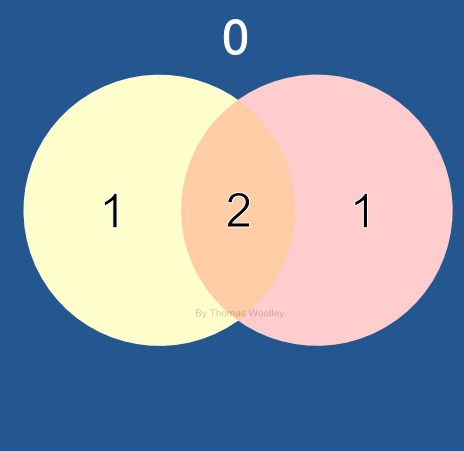For example, in the 2-set Venn diagram, the region outside of the circles has rank zero, because any point out there is in neither set. The sections on the extreme left and right of the circles each have rank 1, because they are a member of the either the right or left sets only. Finally, the overlapping region in the center has rank 2, because this section belongs to both sets.

Using this definition we can now head towards proving the initial statement. We do this by combining three smaller proofs concerning a general $N$-set Venn diagram. Explicitly, we
1. count how many sections there are of each rank;
2. show that in a rotationally symmetric Venn diagram these ranks must be divisible by $N$;
3. prove that the ranks are divisible by $N$ if and only if $N$ is prime.
Without further delay the three theorems and proofs can be found below.

Theorem 1
Suppose $k$ is an integer where $0\leq k\leq N$ then in an $N$-set Venn diagram there are
\begin{equation}
\frac{N!}{k!(N-k)!},
\end{equation}
sections of rank $k$ .

Proof 1
In order to help our intuition in counting the sections of a given rank we consider the 1-, 2-, 3-, 4-set Venn diagrams and summarise their details in the table below.

Number of
sections of
each rank
Rank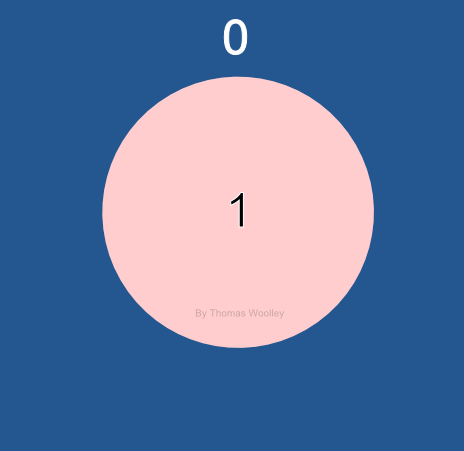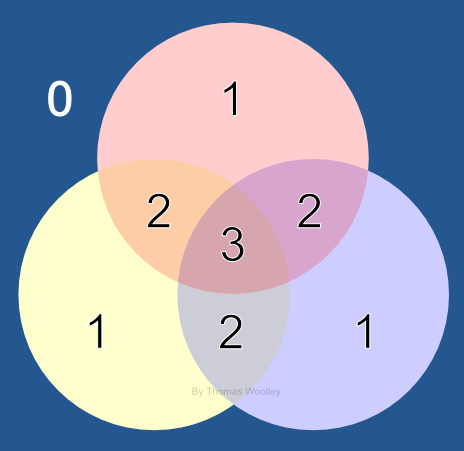01234
Venn
diagram
size
0 sets1

1 set11

2 sets121

3 sets1331
4 sets14641

The pattern of numbers within the table maybe familiar to you, as it is the famous Pascal's triangle. We may have expected these numbers to appear in this formation, because the numbers in Pascal's triangle represent exactly the quantity we are trying to calculate. Namely, the $(k+1)^{th}$ number in the $(N+1)^{th}$ row of Pascal's triangle tells us how many ways there are of choosing $k$ objects from $N$ objects. Note that we need to use the $(k+1)^{th}$ number and not the $k^{th}$ number, because the first number of each row deals with the trivial section, which is in none of the sets. Similarly, we use $(N+1)^{th}$ row and not the $N^{th}$ row because the first row deals the trivial Venn diagram of 0 sets.

To cement this idea, suppose we have three objects {A,B,C}. How many different ways are there of choosing two objects from these three (under the assumption that ordering doesn't matter)? Explicitly, there are three ways, {A,B}, {B,C} and {A,C}. This is exactly the answer we get from Pascal's triangle if we look at the third number on the fourth row. Because of this identification we normally call the numbers $N$ choose $k$, or, $_NC_k$, for short.

There are many ways to calculate the coefficients of Pascal's triangle. The quickest way is to use the formula 
\begin{equation}
_NC_k= \frac{N!}{k!(N-k)!}.
\end{equation}

Theorem 2
If an $N$-set Venn diagram is rotationally symmetric the number of section of a given rank $k$, where $0\leq k\leq N$, must be divisible by $N$.

Proof 2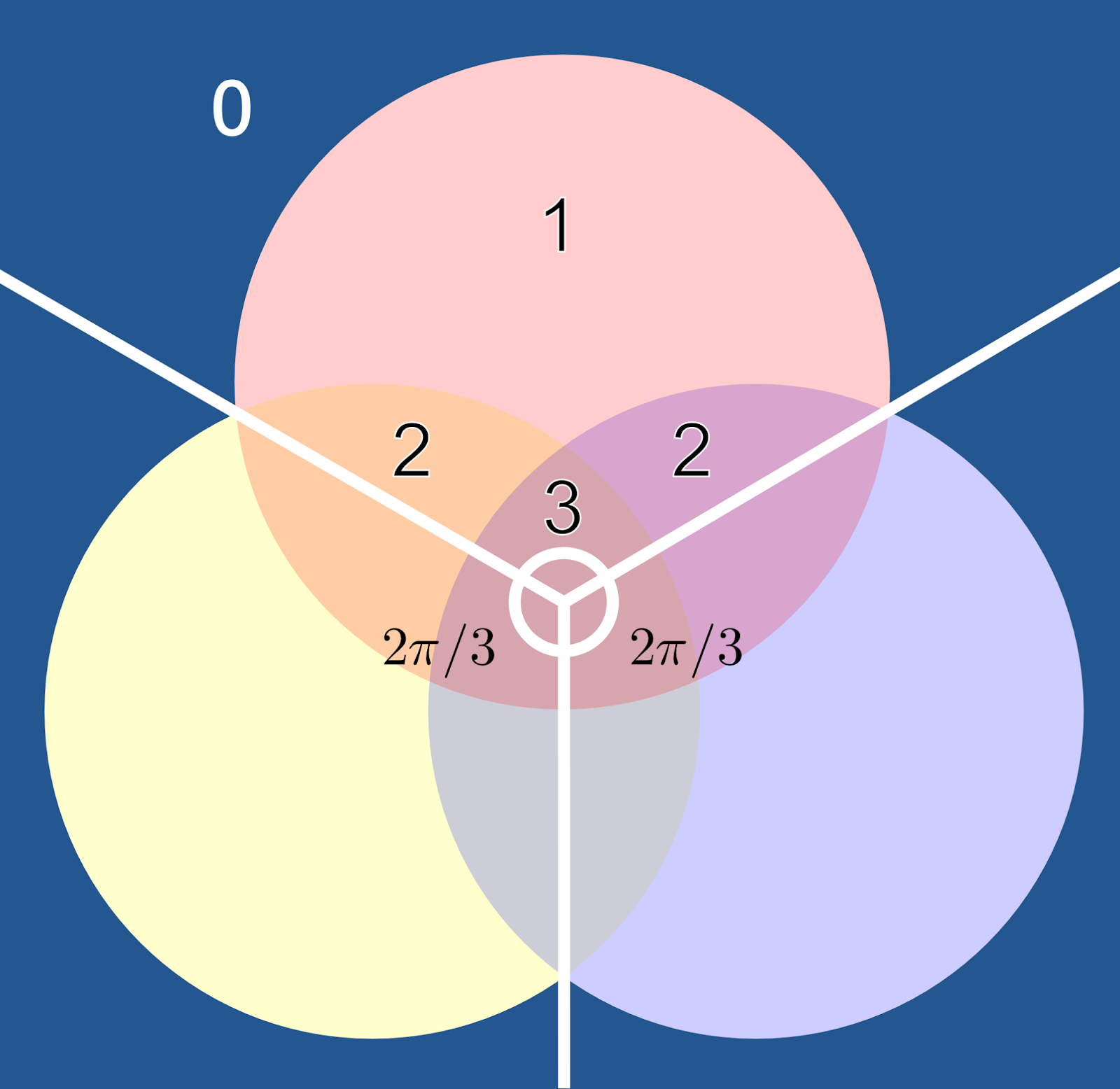By definition, an $N$-set Venn diagram is rotationally symmetric if it has $N$ orders of rotational symmetry. This means that if we find the rotational center of the diagram and split the diagram into equal sectors of $2\pi/N$ radians (or $360/N$ degrees) each sector should "look" identical. For example, have a look at the 3-set Venn diagram which has been split into thirds. Each third contains sections with exactly the same rank.
We deduce that the total sum of sections with rank $k$ (where $0\leq k\leq N$ ) must be divisible by $N$. Suppose it wasn't true, we would not be able to divide the sections of rank $k$ into $N$ identical parts and therefore our diagram could not have $N$ identical sectors, suggesting that the diagram wasn't rotationally symmetric. In order to avert this contradiction we conclude that $N$ divides the total number of sections with rank $k$ for all values of $k$, where $0\leq k\leq N$.

Theorem 3
\begin{equation}
_NC_k= \frac{N!}{k!(N-k)!},
\end{equation}
is divisible by $N$ for all values of $k$ such that $0\leq k\leq N$ if and only if $N$ is prime.

Proof 3
Firstly, suppose $N$ is prime. Consider $N$ choose $k$, which has a simplified form
\begin{equation}
_NC_k= \frac{N(N-1)(N-2)\dots(N-k+1)}{k(k-1)(k-1)\dots1},
\end{equation}
for all  $0\leq k\leq N$. Note $_NC_k$ is an integer and that the top of the fraction has a prime factor $N$, which does not cancel down because there is no factor of $N$ on the bottom. Thus, $_NC_k$ contains a factor of $N$, and so it is divisible by $N$.

Conversely, suppose $N$ is composite. Choose $p$ to be the smallest prime factor of $N$, and define $n=N/p$ then
\begin{equation}
_NC_p=\frac{N(N-1)(N-2)\dots(N-p+1)}{p!}=\frac{n(N-1)(N-2)\dots(N-p+1)}{(p-1)!}.
\end{equation}
Now if $_NC_p$ is divisible by $N$ the top of the fraction must contain a factor of $N$ and therefore a factor of $p$. Because $N=np$ and we already have a factor of $n$ on the top then $p$ must divide at least one of the factors of $N-1$, $N-2$, ..., or $N-p+1$. But $p$ divides $N$, so it does not divide any of these other factors. Once again the only way out of the contradiction is to conclude that when $N$ is composite $N$ does not divide $_NC_p$ and, hence, $_NC_k$ is divisible by $N$ for all values of $k$ such that  $0\leq k\leq N$ if and only if $N$ is prime.

Putting these three theorems together we generate the proof we were originally searching for.

Theorem 4
If $N$ is a composite number then the $N$-set Venn diagram is not rotationally symmetric.

Proof 4
• By proof 1 the total number of sections of a given rank is given by $_NC_k$.
• By proof 2 if a Venn diagram is rotationally symmetric the total number of sections of a given rank must be divisible by $N$ for all $0\leq k\leq N$ .
• We conclude that if a Venn diagram is rotationally symmetric $_NC_k$ must be divisible by $N$ for all  $0\leq k\leq N$.
• Finally, by  proof 3, $_NC_k$ is be divisible by $N$ for all  $0\leq k\leq N$ if and only if $N$ is prime.
Therefore, if $N$ is composite $N$ does not divide $_NC_k$ for all  $0\leq k\leq N$ and, hence, the Venn diagram cannot be rotationally symmetric.

We finish this week's post by noting that, although we have proven that an $N$-set Venn diagram is not rotationally symmetric when $N$ is composite, this does not mean that it is rotationally symmetric if $N$ is prime. We will discuss more about this point next time.
________________________________________________________________
________________________________________

 For those of you who may have forgotten the definition: a positive number is prime if and only if it was only two distinct integer factors, which are itself and 1. For example 2, 3, 5 and 7 are all prime numbers. However, 9 is not a prime number, because 3$\times$3=9 and the factors of 9 are 1, 3 and 9. Any number that is not prime (other than 1) is called composite. The number 1 is a special case as it is neither prime nor composite. It is called a unit.

 The exclamation mark symbol $n!$ represents the product of all positive integers up to and including  $n$, i.e. $n!=1\times2\times3\times...\times n$.

 Ok, I've slightly cheated and not proven that this formula does give us the numbers that we want. However, if you want to read more about the connection between the Pascal numbers and the formula see this post.

 Note that we have excluded $k=0$ and $k=N$, why is that?

## Monday, 8 September 2014

### Back to rotationally symmetric Venn diagrams

Over the past few posts we have been considering Venn diagrams, their properties and their uses. Although we are usually more concerned with what goes into the Venn diagram sets than their actual shape mathematicians like to abstract everything they can get their hands upon. Thus, they very quickly stopped thinking about the things inside the sets and simply began to consider the properties of the diagrams themselves.

An algorithm for creating a Venn diagram with any numbers of sets was quickly found. For example, Anthony Edwards showed that a diagram containing any number of sets can be constructed using symmetric wavy curves as shown in the animation below.Anthony Edwards' construction of a diagram which will contain the intersection of all sets. However, it is not a true Venn diagram as, although all possible intersections do appear, some of the intersections appear more than once.
Having solved the basic problem of showing existence further constraints where added to the problem. In particular, mathematicians asked whether it was possible to create rotationally symmetric Venn diagrams. To be honest, I have no idea why rotational symmetry is so highly prized, other than it quite aesthetically pleasing.

A rotationally symmetric Venn diagram of $N$ sets is simply a Venn diagram that can be rotated around its centre, such that after $360/N$ degrees (or $2\pi/N$ radians) the graph looks the same as it did initially. With small numbers of sets rotationally symmetric Venn diagrams are fairly easy to produce. For example below are $N=$2, 3 and 5 set diagrams.
Unfortunately, we are unable to create a rotationally symmetric Venn diagram with 4 sets. As we have seen previously, the 4 set diagram cannot be created using circles. Instead, ovals can be used, as seen below.
Of course, just presenting one 4 set diagram that is not rotationally symmetric is not a proof that such a representation does not exist. Perhaps there is a 4 set Venn diagram with non-regular shaped sets that is rotationally symmetric? Fortunately, there is a simple proof that shows that only prime number set diagrams could possibly be rotationally symmetric. Next time I will reproduce the proof that for any composite number $N$ the accompanying $N$ set Venn diagram cannot be rotationally symmetric.

## Monday, 25 August 2014

### Dangerous caterpillars solution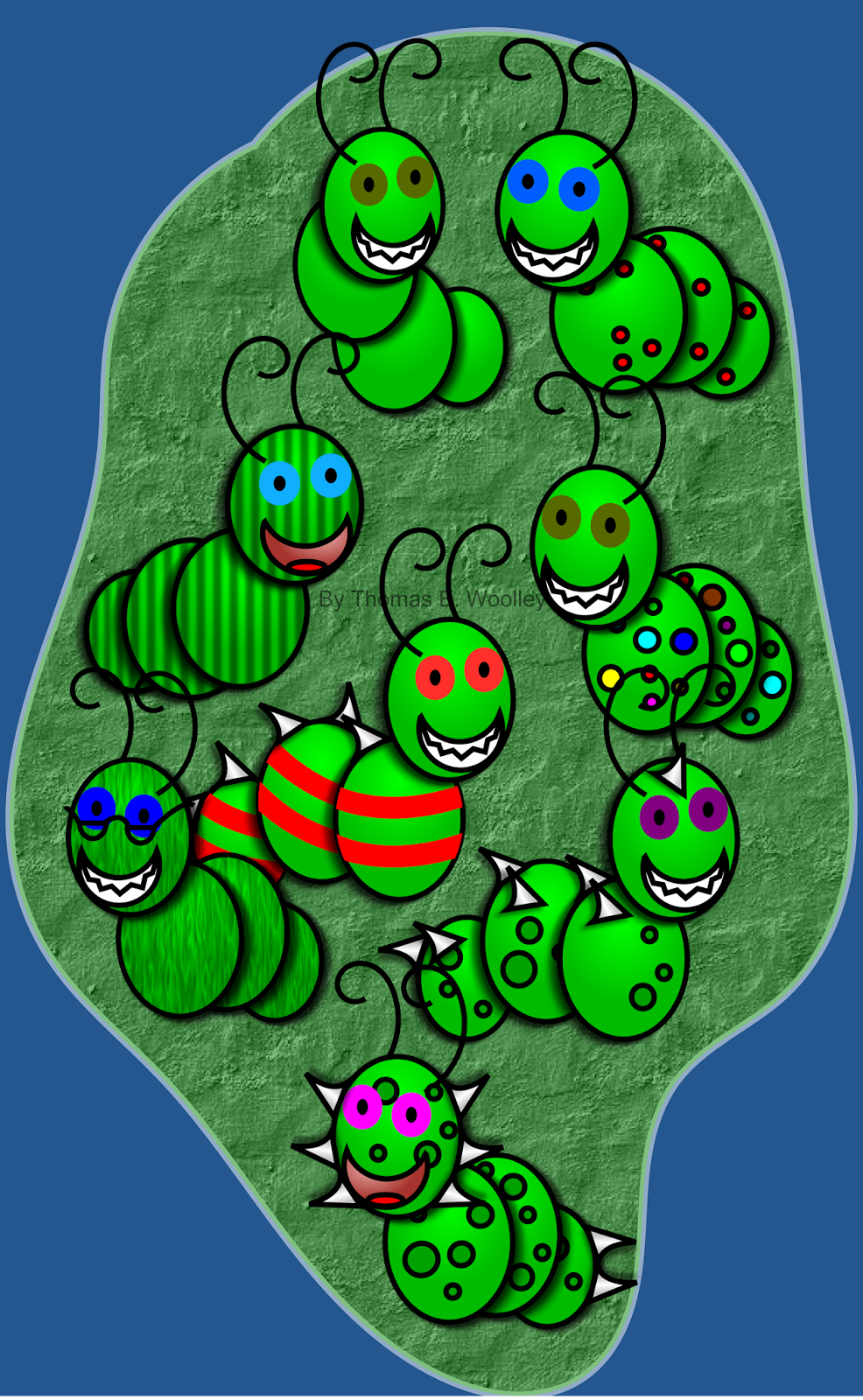Figure 1. The usual suspects.
Last time I asked if we could work out which caterpillars from Figure 1 are safe based on the following rules:
1. If a caterpillar has blue eyes or spots it is dangerous. If a caterpillar has both, we can't tell if it is dangerous.
2. All safe caterpillars have more than one of the following features: teeth, blue eyes, spots, or spikes.
3. Caterpillars with both teeth and spots are dangerous.
As I suggested Venn diagrams are expertly suited to this kind of puzzle.

To solve the puzzle using Venn diagrams we, first, decide what our sets are going to be. In the clues there are 4 features that are discussed: teeth, blue eyes, spots and spikes. Thus, we construct a Venn diagram of these four qualities and insert the caterpillars to their appropriate spaces. This can be seen in Figure 2. Note that in this specific problem we have to use the full Venn diagram form of the four sets, rather than the Euler diagram that we discussed, previously.Figure 2. Separating the caterpillars into types.
Now that we have our diagram we use the clues to remove sections and restrict the safe caterpillar possibilities.

The simplified Venn diagrams corresponding to each of the clues can be seen in Figure 3. For example, the first clue states that animals with blue eyes and spots (but, perhaps, not both) are dangerous. Thus, we remove the sections corresponding to the blue eyed caterpillars and the spots. However, the region over which they intersect is left, because we can't be sure if those caterpillars are dangerous or not.Figure 3. Removing sections of the Venn diagram based on the clues. Left: the blanked out section corresponds to either having blue eyes or spots, but not both. Center: removing the sections with only one feature. Right: removing the section with teeth and spots.
This process is carried out for all three clues. Finally, we put together only the sections that remain in all three diagrams in Figure 3 to get Figure 4.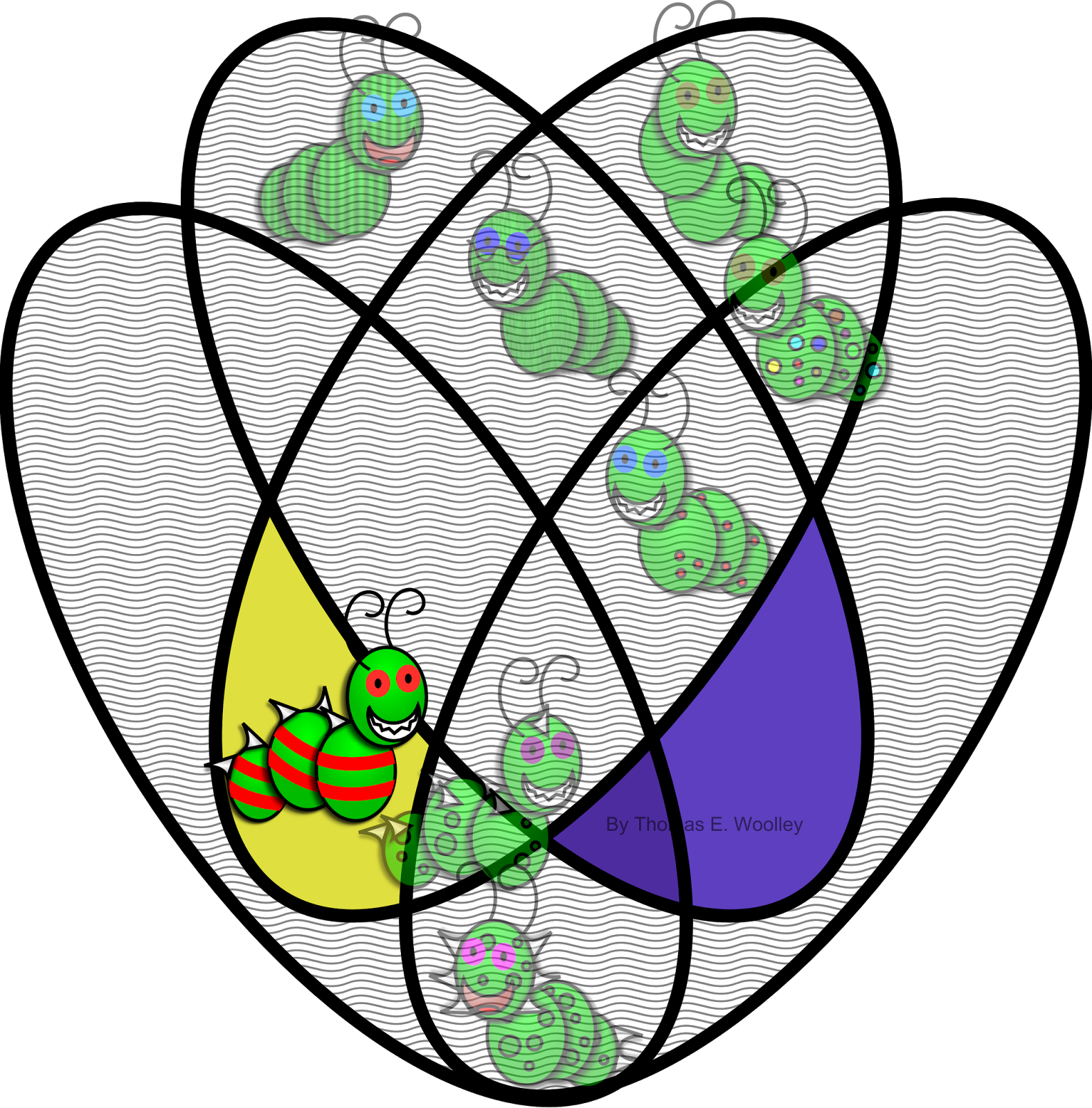Figure 4. Only one caterpillar left.As you see will from Figure 4 there are only 3 sections of the Venn diagram left. These include two sections in the blue eyes and spots region. Because of rule 1, we cannot tell if those sections are safe, or not. Luckily, there are no caterpillars in these regions, so no risks need be taken. The only caterpillar left has both spikes and teeth... would you trust this guy?

## Monday, 11 August 2014

### More Venn diagrams with a logic puzzle

A long time ago I posted about Venn and Euler diagrams and I have been meaning to get back to this subject. Coincidentally, it was John Venn's 180th birthday on the 4th of August, celebrated by Google, so I cannot think of a better time to revive the subject.

Firstly, we recall the all important definition: a Venn diagram contains every single possible intersection between all combinations of the sets. This can be compared with an Euler diagram, which only shows the intersections in which you are interested. An illustration of this can be found in Figure 1.Figure 1. Using four shapes a basic Euler diagram is not the same as a Venn diagram. The right shows an Euler diagram whereas the left is a Venn diagram, as it contains all possible combinations of the four groups.
As I mentioned, these are great ways of seeing logical information quickly and clearly. To show how useful they are let us consider the following puzzle.
________________________________________________________________
________________________________________
I have a collection of 8 caterpillars that have a range of different features. They can all be seen in Figure 2, below.
Unfortunately, some of them are dangerous to touch. Equally troubling is that I do not know which the dangerous ones are! All I know is that the following three statements are true:
1. If a caterpillar has blue eyes or spots it is dangerous. If a caterpillar has both, we can't tell if it is dangerous.
2. All safe caterpillars have more than one of the following features: teeth, blue eyes, spots, or spikes.
3. Caterpillars with both teeth and spots are dangerous.
Using just these facts, which caterpillars are safe to touch?

Now of course there are many ways to solve this little puzzle, but Venn diagrams offer a really nifty way of seeing the solution simply and completely. Have a go at solving it and I'll post the solution next time.

## Monday, 28 July 2014

### Life Saving Mathematics

Heart attacks, cancer, brain tumours and stem cells are all extremely important medical topics. Experimentation in these fields has enabled our knowledge of the human body and its inner workings to increase dramatically during the 20th and 21st century, allowing us to live longer than ever before.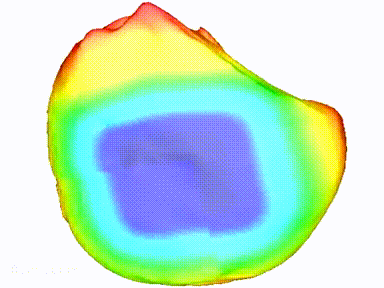Heart fibrillation, a vascularised tumour and a stem cell.

Looking to the future, wouldn’t it be great if we could increase the pace of medical advances? Wouldn’t it be great if we could test possible treatments quickly and cheaply, whilst reducing experimental waste? Wouldn’t it be great if we could predict the most important experiments, to perform before even putting on a lab coat?

Mathematical modelling has the potential not only to meet these requirements but also to do so much more.

Interested in finding out how the subject you hated most is paving a new way in the biomedical sciences? Then join me, Dr Gary Mirams and Prof. Helen Byrne at the British Science festival this September in Birmingham, as we discuss our applications of mathematics and computer science to the pressing medical problems of the 21st Century.Gary, Helen and I will be your hosts for the afternoon.

‘Life saving mathematics’ is scheduled to take place on:
Date: Wednesday 10th September
Time: 13.00 - 14.30
Location: Lecture Theatre W65, Aston Webb Building (180 Capacity)

## Monday, 14 July 2014

### Mathematics through the eyes of the players

2014 marks the 50th anniversary of the Institute of Mathematics and its Applications.
To commemorate this momentous occasion not only did they have the usual conferences and celebratory talks but they also published a book.

50 visions of mathematics is available from the OUP website for a price of £24.99. Think about it… that’s two visions of maths per pound! Who can argue with such a bargain?

Contained within this fully illustrated book you will find 50 chapters of interesting, often cutting edge and, certainly diverse mathematics. All of the articles are written from the personal view points of the authors. The individual and personal tones of the chapters gives them an authentic voice that conveys the excitement and love of the authors and will undoubtedly convince any cynical reader as to the wide ranging power of mathematics.

The authors (whose roster boasts such names as David Acheson, Simon Singh and Ian Stewart) come from many different backgrounds such as: research; teaching and science communication. So, you can be sure that the chapters are written to entertain as well as inform.

I, too, have written a chapter for the book on my favourite subject of Turing patterns. Not only am I excited at the chance to demonstrate their mathematical and visual beauty (blog posts on Turing patterns can be found here and here) to a wide audience, but I have also been immortalised in the pages of the book. When talking about the application of Turing patterns to animal skins (discussed here) I make reference to the ring tailed lemur contradicting the theory. Luckily, I had recently fed some ring tailed lemurs in Newquay zoo and so my picture (a different one below) can now be found in every copy of the book.Myself and Lorraine feeding some very lovely lemurs at Newquay zoo.
Apart from my own ego stroking there are articles concerning the mathematics seen in the recent movie “Sherlock Holmes: A Game of Shadows” written by Derek Moulton and Alain Goriely, who were actually employed by the filmmakers to come up with Moriarty’s codes. There are interesting chapters about the medical applications of mathematics from Richard Elwes and Carson C. Chow. There is even a chapter on the mathematics of murder scenes, which discusses how to calculate the original location of a set of blood splashes.

There are a few chapters that feel a little “in jokey” and not to my taste, including how different sources might quote Pythagoras’ theorem. For example you might see tweets saying
“OMG, for right angled triangle squares on sides add up :) #pythagoras”.
However, these are small details and I can think of no recent brief anthology that can give you a better range of mathematics across history and application. Perhaps, more importantly, the book gives you insights into the people behind the mathematics. It demonstrates that mathematicians are human too. We are interested in using mathematics to make the world a better place and we want to communicate these ideas to people like you.

In summary, you will know if this is book is for you. If you are a recreational scientist interested in the forefront of  mathematics then I happily recommend it.

## Monday, 30 June 2014

### Inpainting: a users’ guide

Over the past few posts I have introduced the idea of inpainting and the mathematics behind the ability to “intelligently” fill in a region based on the information provided by the rest of the image. The theory was developed by Dr Thomas Maerz, from which he constructed a set of matlab codes that simulated his ideas. Dr Martin Robinson has since developed Thomas’ ideas into a FREE GIMP plugin.

This week I present a guide to using Martin’s plugin.
________________________________________________________________
________________________________________
In its most basic form Martin’s code is extremely easy to use: you select a region; set a few parameters and tell GIMP to run the code. As before, we will use the plugin to remove bee from the following photo. Also, we once again use the GIMP’s extensive suite of selection tools to highlight the region we are interested in.
When you have selected the region you are interested in fire up the inpainting plugin. If it has been installed correctly (see Martin’s documentation for more information) then by default it should be in the “Filters” drop down menu under “Misc”. Once it is opened you should see a box that looks like Figure 1.

First approximationFigure 1. The general user interface of the inpainting plugin.
In this basic case the text box should automatically be filled in with the correct details. Namely, the image shown at the top should be the area that you selected, the source should be the image you want to inpaint and the mask type should be a selection.

You will then notice that there are four parameters: epsilon, kappa, sigma and rho. These are very important and control various aspects of the inpainting algorithm. Specifically, not only do they influence what colour a chosen pixel will be, but they also influence how details will propagate into the domain. This means if there are straight lines leading into the boundary the parameters will alter how those straight lines are continued. The following box gives more detail on each of the parameters.

 epsilon defines the region over which the colour is averaged. If epsilon is large then colours over a larger region are used. If epsilon is small the code only uses local colour detail to paint the new pixel. kappa controls how the level set lines (discussed in a previous post) effect the direction of features that we want to propagate into the region. If we want the picture features to follow the level set line direction we make kappa large, otherwise we make it small. sigma controls how much the input data is smoothed. If sigma is small then small scale features will propagate into the domain, alternatively, if sigma is large then only clear, distinct line features will be taken into the inpainting region. rho controls the averaging of the directional information. This is needed because some of the features may be heavily directional and we may want to propagate these directions into the image. A large rho causes a large amount of information of the boundary to be averaged, leading to more accurate directional information. If there is little directional information in the boundary we make rho small.

In the basic case shown below I set the parameters to epsilon=20, kappa=100, sigma=0.01, rho=20. The way I found these numbers was partly through playing around with the sliders and partly thinking about their definitions. Since the picture is high definition we want the maths to take in detail from a large region, so we make epsilon big. Because there are a number of thin creases in the petals that we want to propagate and carry on the line we set kappa and rho high (to keep the direction) and sigma low (to keep the detail).

As you will see below even this simple approach does a good job. However, by putting in a little extra effort we can make the result so much better.
As you may notice from the image, most of the lines meet up one the bee is removed. However, because the bee covers regions connecting the petals to the central anthers of the plant, some of the central plant detail gets propagated into the inpainted region. To stop details being propagated in to regions we do not want them we use a “stop path”.

We select the path tool from the gimp work box and draw a curve. This curve will represent the points to which the details will propagate. Since the anthers are propagating too far we draw a red line that maps closely to the anther region as seen below. As before, the black and white line represents the region selected to be inpainted.
Once we have the path defined we then go back to the plugin and select “Selection With Stop Path” in the “Mask Type” drop down menu. Once this is selected the “Stop Path” drop down menu should become selectable. In this menu we select the curve that we have drawn and, finally, click apply.

Below we show the base case (left) along with the final image of this advanced version (right), and both of these can be compared with the original (above). Hopefully, you can see that, by just applying a little more work we get a much better result.

________________________________________
Over the last few posts I have introduced the idea of inpainting, met the minds behind it and, hopefully, you’ve had ago yourself. Once again, here are all the links that have mentioned over the last few weeks:

## Monday, 16 June 2014

### Inpainting: how does it work?

Last time I introduced the idea of inpainting, which is an automatic way of “intelligently” filling in a region of an image. Dr Thomas Maerz has worked on a technique which is not only computationally quick, but can produce astounding results. Incredibly, Thomas is allowing his ideas to be made freely available by Dr Martin Robinson who has implemented them in a simple to use GIMP plugin, which can be downloaded from here.

This week we take a look at the theory behind the code.

If you are interested in discovering more about the mathematics of inpainting then Thomas' papers can be found here and here, whilst an original version of Thomas' matlab code can be found on github.
________________________________________________________________
________________________________________
1) Select the image.
You begin with an image, in which there is a region you want to remove, or repair. As an example, let’s take the image below and try to remove the bee from the flower.
2) Select the region.
Select the region using the many tools available in gimp such as the rectangle box, colour selection, or, as I used here, the simple free hand tool. You do not have to delete the area, but since the interior is ignored I will delete it to clearly show the region we are working with.
3) Create level sets.
For all of the pixels in the selected area the plugin calculates the nearest distance to the boundary. In the image below the lines represent contours that are the same distance away from the boundary. For example, the black line represents the white pixels that are 10 pixels away from the nearest boundary; the red line represents the white pixels that are 20 pixels away from the nearest boundary, etc. The colour of the lines are for visualisation purposes only and serve no function in the mathematics.

These contours then form what are known as level sets. Essentially this means that a certain function has a constant value on them. In our case the function is the distance to the boundary.
4) Process the curves.
The curves are processed sequentially, working our way in from the boundary. The boundary is the last region of colour before we enter the cleared region. This boundary gives us all the initial data we need and it is propagated into the region.

Since this curve is coloured we step to the next contour, for example the black contour in the above picture. We choose a pixel on this contour and consider all the previously coloured pixels. This new pixel is filled in with a colour value that is a weighted average of the previously coloured pixels. Namely, coloured pixels that are close to the white pixel we are considering are given more weight when it comes to choosing the appropriate colour for the white pixel. Once the colour is chosen we move to the next white pixel on our current contour. Once all the pixels on the contour are coloured we move into the next contour.
This provides gimp with an algorithm allowing it to automatically fill in the white region, as shown above.

5) Enjoy the result.
The bee (in the original image on the left) has been completely removed in the processed image on the right.
________________________________________________________________
________________________________________

Although the final image on the right was produced in approximately 30 seconds it can still be improved upon as there are clearly regions where the creases in the petals do not line up. Next time I will present a user guide to Martin’s implementation of Thomas’ work and show that this can be improved upon.